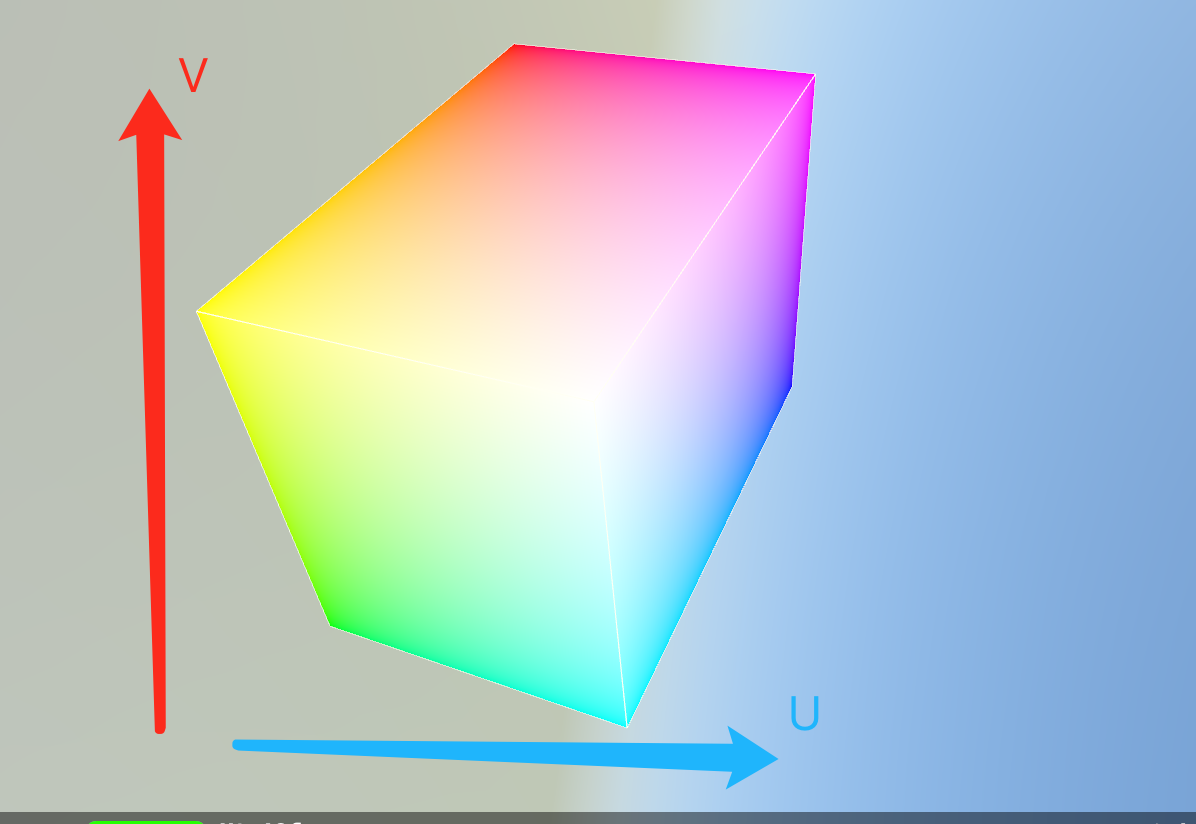# S03E09： RGB 与YUV 转换矩阵的几何含义

## 说明

YCbCr 是在世界数字组织视频标准研制过程中作为ITU - R BT1601 建议的一部分, 其实是YUV经过缩放和偏移的翻版。其中Y与YUV 中的Y含义一致, Cb , Cr 同样都指色彩, 只是在表示方法上不同而已。在YUV 家族中, YCbCr 是在计算机系统中应用最多的成员, 其应用领域很广泛,JPEG、MPEG均采用此格式。一般人们所讲的YUV大多是指YCbCr。

## 几何

YUV 与 RGB 的转换公式不止一种，主要原因是具体格式下，标准不同。本文目的是介绍这个转换的几何意义，所以我们这里采用苹果 Demo 中给出的转换矩阵，其它转换公式中，具体数值可能不同：

``````let ycbcrToRGBTransform = float4x4(
simd_float4(+1.0000, +1.0000, +1.0000, +0.0000),
simd_float4(+0.0000, -0.3441, +1.7720, +0.0000),
simd_float4(+1.4020, -0.7141, +0.0000, +0.0000),
simd_float4(-0.7010, +0.5291, -0.8860, +1.0000)
);

``````R = Y + 1.402*V - 0.701
G = Y - 0.3441*U - 0.7141*V + 0.5291
B = Y + 1.772*U - 0.886

• 将一个 RGB 的颜色用 xyz 坐标表示，那么将这个坐标（旋转、缩放、平移）之后，新的 xyz 坐标就可以表示 YUV 颜色值；
• 反之也是，将一个 YUV 颜色分量当做 xyz 坐标，那么将这个坐标逆向（旋转、缩放、平移）之后，新的 xyz 坐标就可以表示 RGB 颜色值；## 代码

``````let box1 = scene.rootNode.childNode(withName: "box", recursively: true)!
let box2 = scene.rootNode.childNode(withName: "box2", recursively: true)!
simpleProgram(node: box1)
simpleProgram(node: box2)

//YUV 到 RGB
let ycbcrToRGBTransform = float4x4(
simd_float4(+1.0000, +1.0000, +1.0000, +0.0000),
simd_float4(+0.0000, -0.3441, +1.7720, +0.0000),
simd_float4(+1.4020, -0.7141, +0.0000, +0.0000),
simd_float4(-0.7010, +0.5291, -0.8860, +1.0000)
);
let p = ycbcrToRGBTransform.inverse//RGB 到 YUV
box1.simdTransform = p

//        box2.simdTransform = box2.simdTransform * ycbcrToRGBTransform.inverse * box2.simdTransform.inverse

``````//用 shader 进行可视化显示
func simpleProgram(node:SCNNode) {
let program = SCNProgram()
program.vertexFunctionName = "vertexShader"
program.fragmentFunctionName = "fragmentShader"

// 赋值给**SCNGeometry**或者**SCNMaterial**
guard let material = node.geometry?.materials.first else { fatalError() }
material.program = program
}

``````//默认的头文件
#include <metal_stdlib>
using namespace metal;
//与 SceneKit 配合使用时，需要的头文件
#include <SceneKit/scn_metal>

struct VertexInput {
float3 position [[attribute(SCNVertexSemanticPosition)]];
};

struct ColorInOut
{
float4 position [[position]];
float4 color;
};

struct MyNodeData
{
float4x4 modelViewProjectionTransform;
};

// 顶点着色器函数，输出为 ColorInOut 类型，输入为 VertexInput 类型的变量 in，和 MyNodeData 类型的变量指针 scn_node
vertex ColorInOut vertexShader(VertexInput in [[stage_in]], constant MyNodeData& scn_node [[buffer(0)]])
{
ColorInOut out;
// 将模型空间的顶点补全为 float4 类型，进行 MVP 变换
out.position = scn_node.modelViewProjectionTransform * float4(in.position, 1.0);
// 加 0.5，将坐标从[-0.5～0.5]，转换到[0～1] 以代表颜色
out.color = float4(in.position + 0.5, 1);
return out;
}

// 片元着色器函数，输出为 half4，输入为 ColorInOut 类型的变量 in
fragment half4 fragmentShader(ColorInOut in [[stage_in]])
{
return half4(in.color);
}